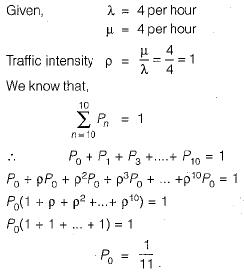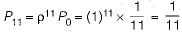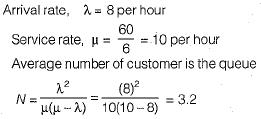Courses

# Test: Queuing Theory - 2

## 10 Questions MCQ Test Topicwise Question Bank for Mechanical Engineering | Test: Queuing Theory - 2

Description
This mock test of Test: Queuing Theory - 2 for Mechanical Engineering helps you for every Mechanical Engineering entrance exam. This contains 10 Multiple Choice Questions for Mechanical Engineering Test: Queuing Theory - 2 (mcq) to study with solutions a complete question bank. The solved questions answers in this Test: Queuing Theory - 2 quiz give you a good mix of easy questions and tough questions. Mechanical Engineering students definitely take this Test: Queuing Theory - 2 exercise for a better result in the exam. You can find other Test: Queuing Theory - 2 extra questions, long questions & short questions for Mechanical Engineering on EduRev as well by searching above.
QUESTION: 1

Solution:
QUESTION: 2

Solution:
QUESTION: 3

### Queuing theory is associated with

Solution:
QUESTION: 4

ln a queuing problem, if the arrivals are completely random, then the probability distribution, of number of arrivals in a given time follows:

Solution:

Number of arrival in queuing problem follow poisson distribution.

QUESTION: 5

Length of the System (Ls) in queuing is equal to

Solution:
QUESTION: 6

Queue Length (Lq) in queuing is equal to

Solution:
QUESTION: 7

In a M/M/1 queue, the service rate is

Solution:

Explanation : Arrivals occur at rate λ according to a Poisson process and move the process from state i to i + 1. Service times have an exponential distribution with rate parameter μ in the M/M/1 queue, where 1/μ is the mean service time.

QUESTION: 8

Patients arrive at a doctor’s clinic according to the Poisson distribution. Check up time by the doctor follows an exponential distribution. If on an average, 9 patients/hr. arrive at the clinic and the doctor takes on an average 5 minutes to check a patient, the number of patients in the queue will be

Solution:
QUESTION: 9

Consider a single server queuing model with Poisson arrivals (λ = 4/hour) and exponential service (μ = 4/hour). The number in the system is restricted to a maximum of 10. The probability that a person who comes in leaves without joining the queue is

Solution:Probability that a person who comes in leaves without joining the queue i.e.QUESTION: 10

The number of customers arriving at a railway reservation counter is Poisson distributed with an arrival rate of eight customers per hour. The reservation clerk at this counter at this counter takes six minutes per customer on an average with an exponentially distributed service time. The average number of the customers in the queue will be

Solution: